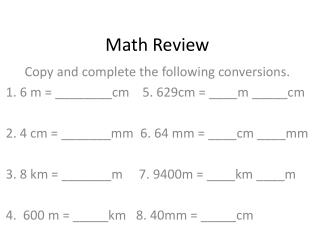# Math Review - PowerPoint PPT PresentationDownload PresentationMath Review

Math Review
Download Presentation## Math Review

- - - - - - - - - - - - - - - - - - - - - - - - - - - E N D - - - - - - - - - - - - - - - - - - - - - - - - - - -
##### Presentation Transcript

1. Math Review Copy and complete the following conversions. 1. 6 m = ________cm 5. 629cm = ____m _____cm 2. 4 cm = _______mm 6. 64 mm = ____cm ____mm 3. 8 km = _______m 7. 9400m = ____km ____m 4. 600 m = _____km 8. 40mm = _____cm

2. Math Review Copy and complete the following conversions. 1. 6 m = __600__cm 5. 629cm = ____m _____cm 2. 4 cm = __40__mm 6. 64 mm = _6_cm _4_mm 3. 8 km = __8 000_m 7. 9400m = _9_km _400_m 4. 600 m = _0.6 or .6 km 8. 40mm = _4__cm

3. Metric Units - Estimation • How tall would you expect the ceiling on Santiago College’s new cafeteria to be? A. 2km B. 80m C. 20 m D. 100cm • How wide would you expect your wrist to be? A. 2mm B. 4 cm C. 80mm D. 1m • How long would you expect our 5C lockers to be? ______________________________________

4. Metric Units - Estimation • How tall would you expect the ceiling on Santiago College’s new cafeteria to be? A. 2km B. 80m C. 20 m D. 100cm • How wide would you expect your wrist to be? A. 2mm B. 4 cm C. 80mm D. 1m • How long would you expect our 5C lockers to be? _____Between 4 and 6 meters long_______

5. What unit? • Write what metric unit you would use to measure the following items. • The height of a book ___________ • The distance from Santiago to Cajon del Maipo ______________ • The distance from center field to the goal on a soccer field ____________ • The width of your ear ___________

6. What unit? • Write what metric unit you would use to measure the following items. • The height of a book _centimeters_____ • The distance from Santiago to Cajon del Maipo__kilometers________ • The distance from center field to the goal on a soccer field __meters_______ • The width of your ear __millimeters______

7. What can you measure? • Write one thing you can measure with each of the following units. • Millimeters _______________________ • Centimeters _______________________ • Meters _______________________ • Kilometers ____________________

8. What can you measure? • Write one thing you can measure with each of the following units. Everyone’s answers will be different. There are many correct answers. A non-example would be – you should not measure distance from Santiago to Mendozza in centimeters. • Millimeters _______________________ • Centimeters _______________________ • Meters _______________________ • Kilometers ____________________